# Daughters

The man conducting the census asks a woman to age of three daughters. Woman says when multiply the age getnumber 72; if their ages add up, get a number of our house, as you see. The man says: That is not enough to calculate their ages. She says: my oldest daughter has a cat with a wooden leg. The man replies: So now I know their age.

How old are her daughter?

Result

x =  3
y =  4
z =  6

#### Solution:The equations have the following integer solutions:
x*y*z = 72
x<y
y<z
s = x+y+z

##### s1=-8, x1=-8, y1=-3, z1=3s2=-7, x2=-6, y2=-4, z2=3s3=-7, x3=-9, y3=-2, z3=4s4=-5, x4=-6, y4=-3, z4=4s5=-2, x5=-6, y5=-2, z5=6s6=-2, x6=-9, y6=-1, z6=8s7=-1, x7=-4, y7=-3, z7=6s8=0, x8=-8, y8=-1, z8=9s9=3, x9=-4, y9=-2, z9=9s10=5, x10=-6, y10=-1, z10=12s11=7, x11=-3, y11=-2, z11=12s12=13, x12=3, y12=4, z12=6s13=13, x13=-4, y13=-1, z13=18s14=15, x14=2, y14=4, z14=9s15=17, x15=2, y15=3, z15=12s16=18, x16=1, y16=8, z16=9s17=19, x17=1, y17=6, z17=12s18=20, x18=-3, y18=-1, z18=24s19=23, x19=1, y19=4, z19=18s20=28, x20=1, y20=3, z20=24s21=33, x21=-2, y21=-1, z21=36s22=39, x22=1, y22=2, z22=36

Number of solutions found: 22

Calculated by our Diofant problems and integer equations.

Leave us a comment of example and its solution (i.e. if it is still somewhat unclear...):

Showing 0 comments:Be the first to comment!#### To solve this example are needed these knowledge from mathematics:

Do you solve Diofant problems and looking for a calculator of Diofant integer equations?

## Next similar examples:

1. Five girls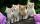Five girls traveling by bus. Each holds in each hand two baskets. In each basket are four cats. Each cat has three kittens. Two girls exits bus. How many legs are in the bus?
2. CagesHonza had three cages (black, silver, gold) and three animals (guinea pig, rat and puppy). There was one animal in each cage. The golden cage stood to the left of the black cage. The silver cage stood on the right of the guinea pig cage. The rat was in the
3. Foot in busIt was 102 people on the bus. 28 girls had two dogs. A 11 girls had one dog. At the next stop seceded 5 dogs (even with their owners). They got two boys together with three dogs. The bus drove one driver. How many foot were in bus?
4. Perpetrator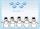The perpetrator is a number that is smaller than the number 80. It is a multiple of five and it is odd. If we added up the tens and ones of this number we get number 8.
5. Decimal expansion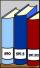Calculate: 2 . 1 + 0 . 10 + 7 . 10000 + 4 . 1000 + 6 . 100 + 0 . 100000 =
6. ProductResult of the product of the numbers 1, 2, 3, 1, 2, 0 is:
7. The largest numberFind the largest integer such that: 1. No figures is not repeat, 2. multiplication of every two digits is odd, 3. addition all digits is odd.
8. SickSick Marcel already taken six tablets, which was a quarter of the total number of pills in the pack. How many pills were in the pack?
9. Multiples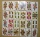What is the sum of the multiples of number 7 that are greater than 30 but less than 56?
10. Travel by bus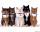Five girls traveling by bus. Each holds in each hand two baskets. Each basket contains 5 cats. Each cat has 5 kittens. 3 girls getting off the bus and another girl without baskets go on board. The bus control driver. How many legs are in the bus?
11. Crocuses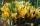The garden grow daffodils, crocuses and roses. 1400 daffodils, crocuses is 462 more and roses is 156 more than crocuses. How many are all the flowers in the garden?
12. Table 3x3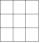Table with numbers 3x3 in the first row 66,24,33 in the second row 57,? 19 in the third row 18,45,60 What number comes ar mark ?. Maybe the numbers: 22,46,45,47
13. Addition of Roman numbersAdded together and write as decimal number: LXVII + MLXIV
14. Street numbersLada came to aunt. On the way he noticed that the houses on the left side of the street have odd numbers on the right side and even numbers. The street where he lives aunt, there are 5 houses with an even number, which contains at least one digit number 6.
15. Combine / add termCombine like terms 4c+c-7c
16. Roman numerals 2+Add up the number writtens in Roman numerals. Write the results as a roman numbers.
17. Roman numerals +Add up the number writtens in Roman numerals. Write the results as a decimal number.